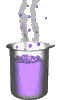Science class online
Chemistry and physics lessons

# Chemistry

Free online chemistry lesson for elementary school, middle school and high school.Science class Chemistry Electricity Optics Mechanics Mechanics lessons Water Water on Earth Changes of state in the nature: the water cycle Water in human body Test for water Properties of water in different states States of matter States of matter and its changes Boiling water Water: freezing and melting Changes of state: mass and volume Molecules in different states of matter Mixtures and solutions Heterogeneous mixtures Homogeneous mixtures Decantation Centrifugation Filtration Vaporization of water Distillation Chromatography Dissolving a solid in water Conservation of mass on dissolving Miscibility in water Mass and volume Volume and its units Measuring volume with a graduated cylinder Mass and its units Measuring the mass of a liquid Mass of a liter of waterVolumetric mass density Air and atmosphere Earth's atmosphere protect us Pressure Atoms and molecules Molecules Molecules in mixtures and pure substances Molecules and states of matter Atoms Composition of molecules Constituents of the atoms The electrical neutrality of the atom Combustions Basics of combustion The combustion of carbon The combustion of butane Atoms and chemical reactions Chemical reactions Chemical equations Law of conservation of mass Chemical synthesis Metals Corrosion of metals Electrical conductivity of solid materials Electrons and free electrons Electric current in metals Ions The conductivity of aqueous solutions Aqueous solutions and ions The direction of movement of ions Formation of ions Tests for ions Acidic and basic solutions pH of aqueous solutions Ions in acidic and basic solutions Dilution of acids and bases Composition of hydrochloric acid Chemical reaction between iron and hydrochloric acid Electrochemical cells and chemical energy Chemical reaction beteween a copper sulphate solution and zinc Copper sulfate and zinc battery Basics of electrochemical cell

 _____________________________________________________   _____________________________________________________

## Mass and volume

### Volume and its units

1) What is volume ?

The volume corresponds to the space occupied by an object or substance (solid, liquid or gaseous).

The volume can be calculated for simple geometric shapes such as
- Cubes: Volume = side x side x side
- Parallelepiped: Volume = length x width x height
as well as spheres, cones, pyramids etc..

For objects of more complex shapes, we must make measurements to determine the volume

2) Units of volum: cubic meter

The standard unif of volume is the cubic meter ( symbol m3 ).
A cubic meter corresponds to the space occupied by a cube of side 1 m.

L'unité de volume du système international est le mètre cube de symbole m
3
Un mètre cube correspond à l'espace qu'occupe un cube de 1 m de coté.

The volume can also be expressed using multiples of the cubic meter:
- The cubic decameter (da
m3)
- The cubic hectometer (h
m3)
- The cubic kilometers (k
m3)
Or using submultiples of the cubic meter:
- The cubic decimeter (d
m3)
- The cubic centimeter (c
m3)
- The cubic millimeter (m
m3)

Each unit is a thousand times greater than the previous one:
1 cubic centimeter is equal to 1000 cubic millimeter.
1 cubic decimeter is equal to 1000 cubic centimeter.
1 cubic meter is equal to 1000 cubic decimeter.
etc.

Each quantity expressed in one of these units can also be expressed in any of the other. This change of units can be achieved using a conversion table:

 km3 hm3 dam3 m3 dm3 cm3 mm3

______________________________________

______________________________________

3) Units of volum: litre

For liquids and gases, it is common practice to use as unit volume litre (L) and its derived units:
- Millilitre (mL)
- Centilitre (cL)
- Decilitre (dL)
- Decalitre (dal)
- Hectolitre (hl)
- Kilolitre (kL)

Each of these units is ten times greater than the previous one:
1 centilitre is equal to 10 ten millilitre.
1 deciliter is equal to 10 centilitre.
A litre is equal to 10 decilitre.
etc.

Each volume expressed in one of these units can be converted to another unit using the following conversion table:

 kL hL daL L dL cL mL

4) Cubic meter and litre

A litre is a unit that was defined as being equal to one cubic decimeter:
1 L = 1 d
m3
Therefore we have also the following equality:
1 mL = 1 c
m3

______________________________________

______________________________________

____________________________________

____________________________________